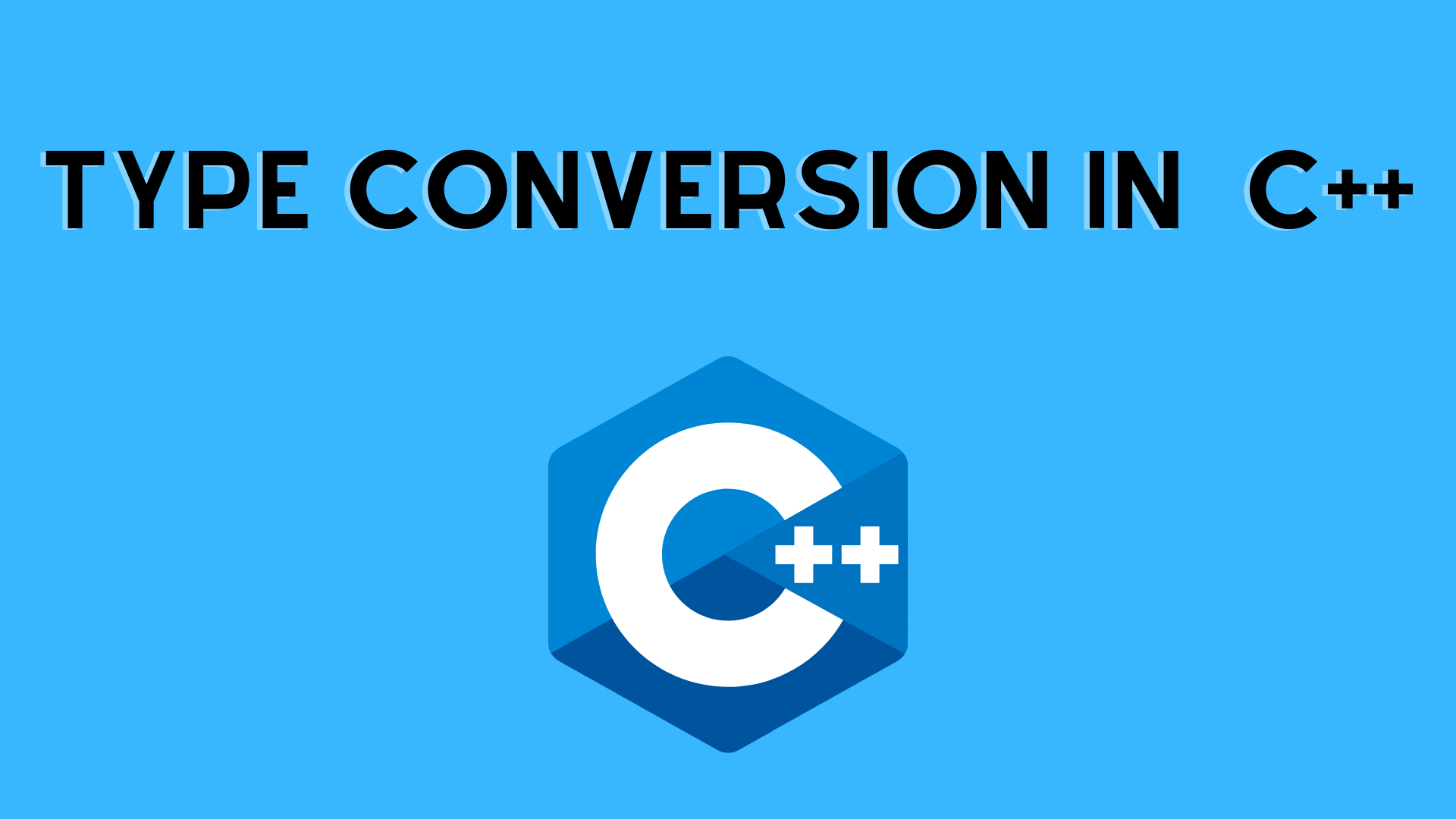## Type Conversion In C++ Programming

We can transform data from one type to another using C++. Type conversion is the term for this.

In C++, there are two types of type conversion.

1. Implicit Conversion(The conversion that isn’t explicit)
2. Conversion Explicit (also known as Type Casting)

### Conversion of Implicit Types

Implicit type conversion refers to type conversion that is performed automatically by the compiler. Automatic conversion is another name for this type of conversion.

• Without any external trigger from the user, the compiler performs this task on its own.
• When more than one data type is present in an expression, this usually happens. To avoid data loss, type conversion (type promotion) occurs in this situation.
• All of the variables’ data types are upgraded to the data type of the variable with the most data.

Implicit conversions can lose data, signs can be lost (when signed is implicitly converted to unsigned), and overflow can occur (when long long is implicitly converted to float).

Let’s have a look at two implicit type conversion instances.

1. Converting an int to a double
```// Working of implicit type-conversion

#include <iostream>
using namespace std;

int main() {
// assigning an int value to i
int i = 8;

// declaring a double type variable
double d;

// implicit conversion
// assigning int value to a double variable
d = i;

cout << "num int = " << i << endl;
cout << "num double = " << d << endl;

return 0;
}```

Output:

num int =8
num double = 8

2. Conversion from double to integer is done automatically.

```//Working of Implicit type-conversion

#include <iostream>
using namespace std;

int main() {

int i;
double d = 8.69;

// implicit conversion
// assigning a double value to an int variable
i = d;

cout << "int = " << i << endl;
cout << "double = " << d << endl;

return 0;
}```

Output:

int = 8
double = 8.69

During the conversion, data is lost (Narrowing Conversion)
As the above example shows, converting from one data type to another might result in data loss. When data of a bigger type is transformed to data of a smaller type, something occurs.

### Explicit Conversion in C++

Explicit conversion occurs when a user explicitly converts data from one kind to another. Typecasting is another term for this type of conversion.

In C++, we can utilize explicit conversion in three different methods. They are as follows:

1. Casting in the C style (also known as cast notation)
2. Function notation (also known as old C++ style type casting) is a method of expressing functions.
3. Type conversion operators

1. Casting for C-style Type

• The C programming language favors this form of casting, as the name implies. Cast notation is another name for it.

This style’s syntax is as follows:

(datatype)expression;

```// initializing int variable
int i = 26;

// declaring double variable
double d;

// converting from int to double
d = (double)i;```

2. Function-style Casting

We can also cast data from one type to another using function like notation.

This style’s syntax is as follows:

datatype(expression);

```// initializing int variable
int i = 26;

// declaring double variable
double d;

// converting from int to double
d = double(i);```

Example: Type Casting

```#include <iostream>

using namespace std;

int main() {
// initializing a double variable
double d = 4.36;
cout << "d = " << d << endl;

// C-style conversion from double to int
int i1 = (int)d;
cout << "i1   = " << i1 << endl;

// function-style conversion from double to int
int i2 = int(d);
cout << "i2   = " << i2 << endl;

return 0;
}```

Output

d = 4.36
i1 = 4
i2 = 4

## Operators for converting types

Aside from these two type castings, C++ contains four type conversion operators. Type conversion operators are what they’re called. They are as follows:

1. static_cast
2. dynamic_cast
3. const_cast
4. reinterpret_cast

You may like:

Input and Output in C++ | C++ Programming

Data Types in C++

Operators In C++ Programming